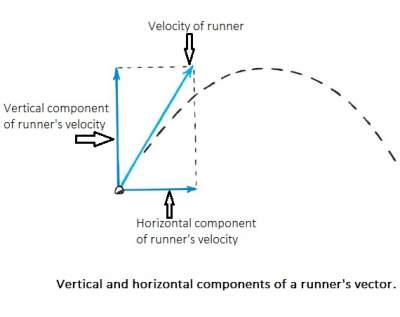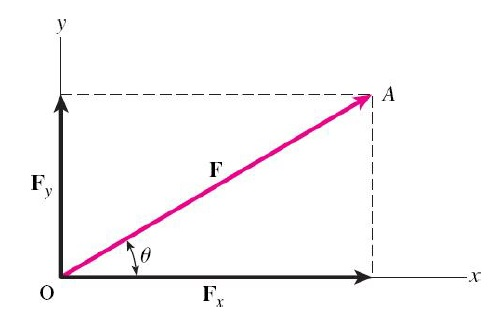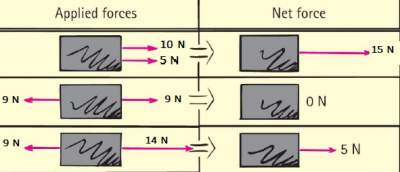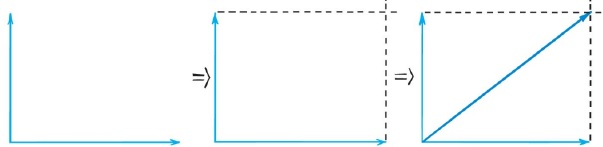# Resultant Force

## Vector Components

Any vector can be resolved into two components perpendicular to each other.
The two components are usually called vertical (referring to the y axis) and horizontal (referring to the x axis).Trigonometry can be used to find the vertical and horizontal components of a vector as shown below:### Example

Question: What are the x and y components of a force of 200 N at an angle of 70°?
Tip: Draw the vector diagram and use trigonometry to find the components.
Solution: Place the tail of the 200-N vector at the origin and indicate the components as shown in the figure.
Solution: Average speed exercise

## Net Force and Vectors

A force is a push or a pull.
Force is a vector quantity. When more than a single force acts on an object, we consider the net force.
The net force is called resultant as well.As one can see, adding vectors that act along parallel directions to find the resultant is simple:
• They add if they act in the same direction.
• They subtract if they act in opposite directions.
To find the resultant of two vectors that don’t act in exactly the same or opposite direction,
we use the parallelogram rule. Construct a parallelogram in which the two vectors are adjacent sides.
The diagonal of the parallelogram shows the resultant. In the figure below, the parallelograms are rectangles.
The pair of vectors at right angles to each other make two sides of a rectangle, and hence the diagonal of which is their resultant as illustrated below:### Exercise

Exercise on Kinetic Energy